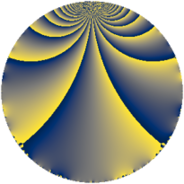# Properties

 Label 4560.2.a.bkLevel $4560$ Weight $2$ Character orbit 4560.a Self dual yes Analytic conductor $36.412$ Analytic rank $1$ Dimension $2$ CM no Inner twists $1$

# Related objects

## Newspace parameters

 Level: $$N$$ $$=$$ $$4560 = 2^{4} \cdot 3 \cdot 5 \cdot 19$$ Weight: $$k$$ $$=$$ $$2$$ Character orbit: $$[\chi]$$ $$=$$ 4560.a (trivial)

## Newform invariants

 Self dual: yes Analytic conductor: $$36.4117833217$$ Analytic rank: $$1$$ Dimension: $$2$$ Coefficient field: $$\Q(\sqrt{5})$$ Defining polynomial: $$x^{2} - x - 1$$ Coefficient ring: $$\Z[a_1, \ldots, a_{7}]$$ Coefficient ring index: $$2$$ Twist minimal: no (minimal twist has level 2280) Fricke sign: $$1$$ Sato-Tate group: $\mathrm{SU}(2)$

## $q$-expansion

Coefficients of the $$q$$-expansion are expressed in terms of $$\beta = \sqrt{5}$$. We also show the integral $$q$$-expansion of the trace form.

 $$f(q)$$ $$=$$ $$q + q^{3} - q^{5} + ( -1 - \beta ) q^{7} + q^{9} +O(q^{10})$$ $$q + q^{3} - q^{5} + ( -1 - \beta ) q^{7} + q^{9} + ( 1 + \beta ) q^{11} + ( -1 + \beta ) q^{13} - q^{15} -2 \beta q^{17} + q^{19} + ( -1 - \beta ) q^{21} + ( -2 + 2 \beta ) q^{23} + q^{25} + q^{27} + ( -5 + \beta ) q^{29} -4 q^{31} + ( 1 + \beta ) q^{33} + ( 1 + \beta ) q^{35} + ( -3 - \beta ) q^{37} + ( -1 + \beta ) q^{39} + ( -3 + 3 \beta ) q^{41} + ( -1 - \beta ) q^{43} - q^{45} + ( -2 + 2 \beta ) q^{47} + ( -1 + 2 \beta ) q^{49} -2 \beta q^{51} + ( -4 - 2 \beta ) q^{53} + ( -1 - \beta ) q^{55} + q^{57} + ( 6 - 2 \beta ) q^{59} -2 \beta q^{61} + ( -1 - \beta ) q^{63} + ( 1 - \beta ) q^{65} + ( 2 - 2 \beta ) q^{67} + ( -2 + 2 \beta ) q^{69} + ( 4 - 4 \beta ) q^{71} + ( 4 + 2 \beta ) q^{73} + q^{75} + ( -6 - 2 \beta ) q^{77} + ( 4 + 4 \beta ) q^{79} + q^{81} + 2 \beta q^{85} + ( -5 + \beta ) q^{87} + ( -11 + 3 \beta ) q^{89} -4 q^{91} -4 q^{93} - q^{95} + ( -7 - 5 \beta ) q^{97} + ( 1 + \beta ) q^{99} +O(q^{100})$$ $$\operatorname{Tr}(f)(q)$$ $$=$$ $$2q + 2q^{3} - 2q^{5} - 2q^{7} + 2q^{9} + O(q^{10})$$ $$2q + 2q^{3} - 2q^{5} - 2q^{7} + 2q^{9} + 2q^{11} - 2q^{13} - 2q^{15} + 2q^{19} - 2q^{21} - 4q^{23} + 2q^{25} + 2q^{27} - 10q^{29} - 8q^{31} + 2q^{33} + 2q^{35} - 6q^{37} - 2q^{39} - 6q^{41} - 2q^{43} - 2q^{45} - 4q^{47} - 2q^{49} - 8q^{53} - 2q^{55} + 2q^{57} + 12q^{59} - 2q^{63} + 2q^{65} + 4q^{67} - 4q^{69} + 8q^{71} + 8q^{73} + 2q^{75} - 12q^{77} + 8q^{79} + 2q^{81} - 10q^{87} - 22q^{89} - 8q^{91} - 8q^{93} - 2q^{95} - 14q^{97} + 2q^{99} + O(q^{100})$$

## Embeddings

For each embedding $$\iota_m$$ of the coefficient field, the values $$\iota_m(a_n)$$ are shown below.

For more information on an embedded modular form you can click on its label.

Label $$\iota_m(\nu)$$ $$a_{2}$$ $$a_{3}$$ $$a_{4}$$ $$a_{5}$$ $$a_{6}$$ $$a_{7}$$ $$a_{8}$$ $$a_{9}$$ $$a_{10}$$
1.1
 1.61803 −0.618034
0 1.00000 0 −1.00000 0 −3.23607 0 1.00000 0
1.2 0 1.00000 0 −1.00000 0 1.23607 0 1.00000 0
 $$n$$: e.g. 2-40 or 990-1000 Significant digits: Format: Complex embeddings Normalized embeddings Satake parameters Satake angles

## Atkin-Lehner signs

$$p$$ Sign
$$2$$ $$1$$
$$3$$ $$-1$$
$$5$$ $$1$$
$$19$$ $$-1$$

## Inner twists

This newform does not admit any (nontrivial) inner twists.

## Twists

By twisting character orbit
Char Parity Ord Mult Type Twist Min Dim
1.a even 1 1 trivial 4560.2.a.bk 2
4.b odd 2 1 2280.2.a.m 2
12.b even 2 1 6840.2.a.bc 2

By twisted newform orbit
Twist Min Dim Char Parity Ord Mult Type
2280.2.a.m 2 4.b odd 2 1
4560.2.a.bk 2 1.a even 1 1 trivial
6840.2.a.bc 2 12.b even 2 1

## Hecke kernels

This newform subspace can be constructed as the intersection of the kernels of the following linear operators acting on $$S_{2}^{\mathrm{new}}(\Gamma_0(4560))$$:

 $$T_{7}^{2} + 2 T_{7} - 4$$ $$T_{11}^{2} - 2 T_{11} - 4$$ $$T_{13}^{2} + 2 T_{13} - 4$$ $$T_{17}^{2} - 20$$

## Hecke characteristic polynomials

$p$ $F_p(T)$
$2$ $$T^{2}$$
$3$ $$( -1 + T )^{2}$$
$5$ $$( 1 + T )^{2}$$
$7$ $$-4 + 2 T + T^{2}$$
$11$ $$-4 - 2 T + T^{2}$$
$13$ $$-4 + 2 T + T^{2}$$
$17$ $$-20 + T^{2}$$
$19$ $$( -1 + T )^{2}$$
$23$ $$-16 + 4 T + T^{2}$$
$29$ $$20 + 10 T + T^{2}$$
$31$ $$( 4 + T )^{2}$$
$37$ $$4 + 6 T + T^{2}$$
$41$ $$-36 + 6 T + T^{2}$$
$43$ $$-4 + 2 T + T^{2}$$
$47$ $$-16 + 4 T + T^{2}$$
$53$ $$-4 + 8 T + T^{2}$$
$59$ $$16 - 12 T + T^{2}$$
$61$ $$-20 + T^{2}$$
$67$ $$-16 - 4 T + T^{2}$$
$71$ $$-64 - 8 T + T^{2}$$
$73$ $$-4 - 8 T + T^{2}$$
$79$ $$-64 - 8 T + T^{2}$$
$83$ $$T^{2}$$
$89$ $$76 + 22 T + T^{2}$$
$97$ $$-76 + 14 T + T^{2}$$